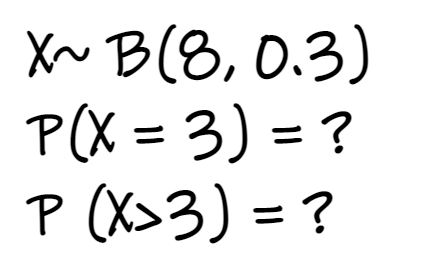# How to find probabilities for binomial distribution with TI 84 graphic calculatorLet’s look at how to find probabilities for binomial distribution with TI 94 graphic calculator, statistics section of H2 A Level Math exam.

For TI 84 graphic calculator, you will make use of the functions: binomcdf and binompdf of your graphic calculator to find the probabilities for binomial distribution.

## How to find binomial distribution probabilities using binocdf and binompdf from TI 84

### To find binompdf

Step 1: Press [2nd] followed by [Vars] to go to [Distr]

Step 2: Use the arrows (up and down) to look for binompdf (it should be “A”), and press enter

Step 3: Once you are at binompdf, you will be asked for 3 inputs: n, p and x.

Step 4: Once the 3 inputs have been typed in, go to paste, and press enter.

==> the probability will be given.

### To find binomcdf

Step 1: Press [2nd] followed by [Vars] to go to [Distr]

Step 2: Use the arrows (up and down) to look for binomcdf (it should be “B”), and press enter

Step 3: Once you are at binomcdf, you will be asked for 3 inputs: n, p and x.

Step 4: Once the 3 inputs have been typed in, go to paste, and press enter.

==> the probability will be given.

## Binompdf vs Binomcdf

When do you use binompdf and when do you use binomcdf?

Binompdf is used when you are finding the probability for a particular value of x. i.e. P(X = x).

Binomcdf is used when you are finding the probability for P(X x). Do note that for binomcdf, it is for values of x lesser than and EQUAL to x.

## Example questions on finding probabilities for binomial distribution with Ti 84

Let’s look at some questions on how to find the probabilities for binomial distribution with TI-84 with some questions. The solutions to the questions can be found here.

Example 1:

Example 2:

## Answers to questions on finding probabilities for binomial distribution with Ti 84

Here’s the answers to the questions on finding probabilities for binomial distribution. Click here to go back to the questions.

Example 1 involves the use of binompdf to find the probabilities for binomial distribution with TI 84.

Example 1 (i) 0.0576 (ii) 0.254

Example 2 involves mainly the use of binomcdf to find the probabilities for binomial distribution with TI 84.

Example 2 (i) 0.806 (ii) 0.552 (iii) 0.0580 (iv) 0.194 (v) 0.942 (vi) 0.254 (vii) 0.551 (viii) 0.390 (ix) 0.687

All the above answers are rounded off to 3 significant figures, which is what’s required for H2 A Level Math exam.

## A Complete Course on H2 A Level Math Statistics

Want a complete course on H2 A Level Math Statistics?

Check out our course here, where we cover all the topics tested in statistics.

The course includes concepts, types of questions, and how to apply them, so that you’ll score for your exam.

Watch a sample video from our course here:

## More on H2 A Level Statistics

• Find a summary of the formulae here
• Find past A Level Papers worked solutions for the various topics here:

permutation and combination

probability

discrete random variables

binomial distribution

normal distribution

sampling

hypothesis testing

linear regression and correlation

error: Content is protected !!